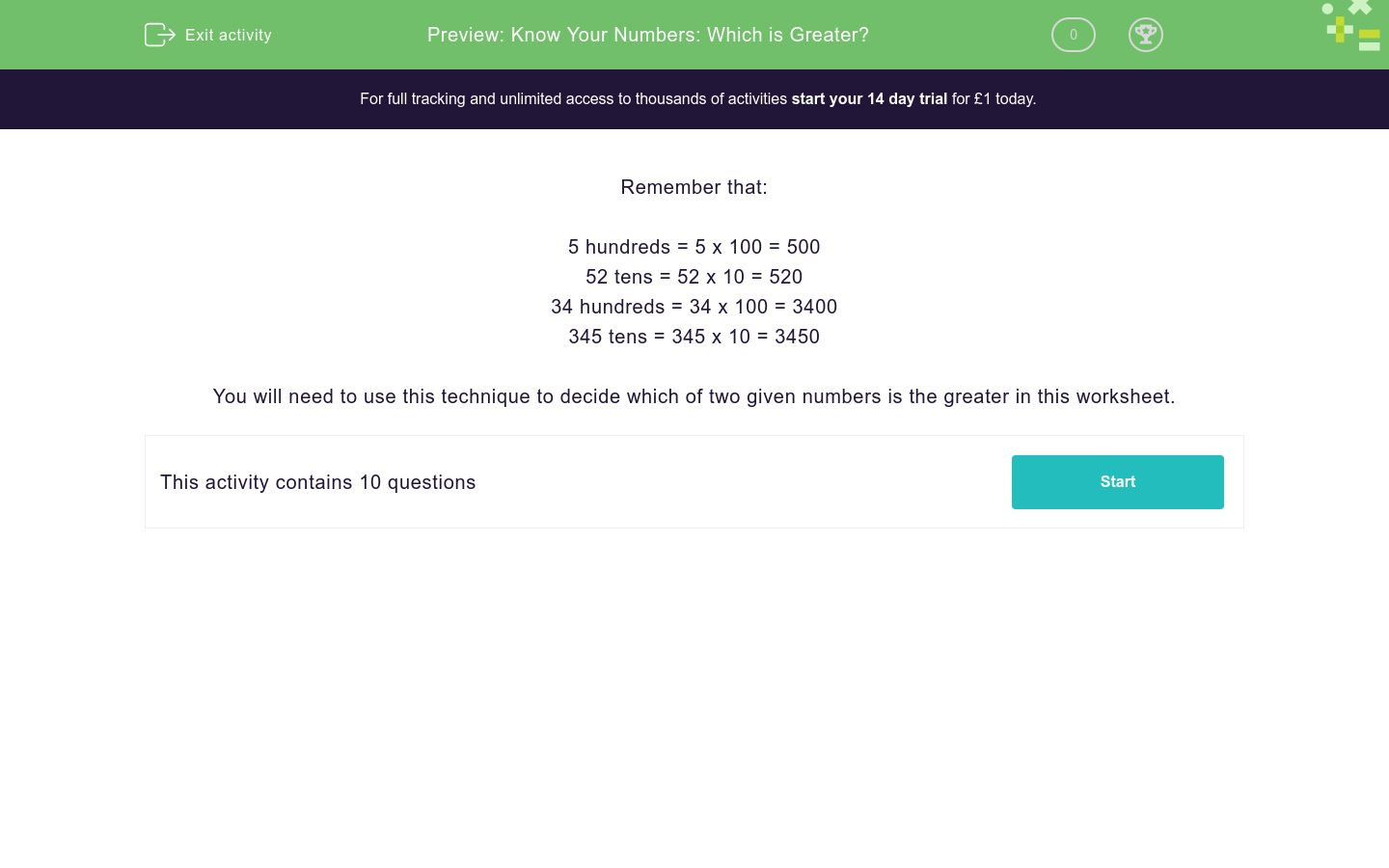# Know Your Numbers: Which is Greater?

In this worksheet, students decide which of two numbers given in different formats is bigger.Key stage:  KS 2

Curriculum topic:   Number: Number and Place Value

Curriculum subtopic:   Know Numbers to 1 000 000

Difficulty level:### QUESTION 1 of 10

Remember that:

5 hundreds = 5 x 100 = 500

52 tens = 52 x 10 = 520

34 hundreds = 34 x 100 = 3400

345 tens = 345 x 10 = 3450

You will need to use this technique to decide which of two given numbers is the greater in this worksheet.

Which of these two numbers is greater?

A.  5 hundreds

B.  45 tens

Choose between A or B or state if they are both the same.

A

B

both the same

Which of these two numbers is greater?

A.  7 hundreds

B.  85 tens

Choose between A or B or state if they are both the same.

A

B

both the same

Which of these two numbers is greater?

A.  9 hundreds

B.  90 tens

Choose between A or B or state if they are both the same.

A

B

both the same

Which of these two numbers is greater?

A.  9 thousands

B.  920 tens

Choose between A or B or state if they are both the same.

A

B

both the same

Which of these two numbers is greater?

A.  6 thousands

B.  57 hundreds

Choose between A or B or state if they are both the same.

A

B

both the same

Which of these two numbers is greater?

A.  60 thousands

B.  60 hundreds

Choose between A or B or state if they are both the same.

A

B

both the same

Which of these two numbers is greater?

A.  60 thousands

B.  6100 tens

Choose between A or B or state if they are both the same.

A

B

both the same

Which of these two numbers is greater?

A.  30 thousands

B.  3000 tens

Choose between A or B or state if they are both the same.

A

B

both the same

Which of these two numbers is greater?

A.  310 thousands

B.  3100 tens

Choose between A or B or state if they are both the same.

A

B

both the same

Which of these two numbers is greater?

A.  37 hundreds

B.  370 tens

Choose between A or B or state if they are both the same.

A

B

both the same

• Question 1

Which of these two numbers is greater?

A.  5 hundreds

B.  45 tens

Choose between A or B or state if they are both the same.

A
EDDIE SAYS
5 x 100 = 500 45 x 10 = 450 500 > 450
• Question 2

Which of these two numbers is greater?

A.  7 hundreds

B.  85 tens

Choose between A or B or state if they are both the same.

B
EDDIE SAYS
7 x 100 = 700 85 x 10 = 850 850 > 700
• Question 3

Which of these two numbers is greater?

A.  9 hundreds

B.  90 tens

Choose between A or B or state if they are both the same.

both the same
EDDIE SAYS
9 x 100 = 900 90 x 10 = 900 900 = 900
• Question 4

Which of these two numbers is greater?

A.  9 thousands

B.  920 tens

Choose between A or B or state if they are both the same.

B
EDDIE SAYS
9 x 1000 = 9000 920 x 10 = 9200 9200 > 9000
• Question 5

Which of these two numbers is greater?

A.  6 thousands

B.  57 hundreds

Choose between A or B or state if they are both the same.

A
EDDIE SAYS
6 x 1000 = 6000 57 x 100 = 5700 6000 > 5700
• Question 6

Which of these two numbers is greater?

A.  60 thousands

B.  60 hundreds

Choose between A or B or state if they are both the same.

A
EDDIE SAYS
60 x 1000 = 60000 60 x 100 = 6000
• Question 7

Which of these two numbers is greater?

A.  60 thousands

B.  6100 tens

Choose between A or B or state if they are both the same.

B
EDDIE SAYS
60 x 1000 = 60000 6100 x 10 = 61000 61000 > 60000
• Question 8

Which of these two numbers is greater?

A.  30 thousands

B.  3000 tens

Choose between A or B or state if they are both the same.

both the same
EDDIE SAYS
30 x 1000 = 30000 3000 x 10 = 30000 30000 = 30000
• Question 9

Which of these two numbers is greater?

A.  310 thousands

B.  3100 tens

Choose between A or B or state if they are both the same.

A
EDDIE SAYS
310 x 1000 = 310000 3100 x 10 = 31000 310000 > 31000
• Question 10

Which of these two numbers is greater?

A.  37 hundreds

B.  370 tens

Choose between A or B or state if they are both the same.

both the same
EDDIE SAYS
37 x 100 = 3700 370 x 10 = 3700 3700 = 3700
---- OR ----

Sign up for a £1 trial so you can track and measure your child's progress on this activity.

### What is EdPlace?

We're your National Curriculum aligned online education content provider helping each child succeed in English, maths and science from year 1 to GCSE. With an EdPlace account you’ll be able to track and measure progress, helping each child achieve their best. We build confidence and attainment by personalising each child’s learning at a level that suits them.

Get started